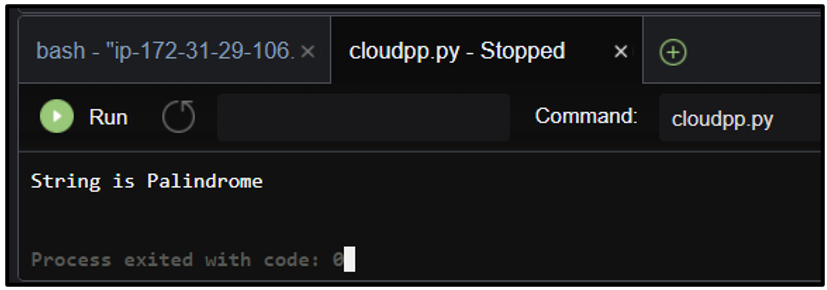top of page
Search

# AWS Cloud9 IDE to develop Python Programs

Learn to use AWS Cloud9 IDE to develop programs in Python language Objective: 1. Learn to use the AWS Cloud9 environment to create various python programs. Step 1: Create a Cloud9 environment. You can refer to our stepwise blog here for creating a Cloud9 environment. Follow step 1 to step 3.

Program 1: Write a program to Reverse a Number.

num = 12345 num_len = len(str(num))

while num_len>0: print(num_len, end = '')

num_len -= 1Program 2. Write a program to check if the string is Palindrome or not. def isPalindrome(s):

return s == s[::-1] s = "radar" ans = isPalindrome(s)

if ans: print("String is Palindrome")

else: print("String is not Palindrome")Program 3. Write a program to check if the number is prime number or not.

num = 14 if num == 1 or num == 2 or num ==3 or num == 5 or num == 7:

print(str(num)+" is Prime number.") elif ((num%3 ==0) or (num%5==0) or (num%7==0)):

print(str(num)+" is not Prime number.") else: print(str(num)+" is Prime number.")Program 4. Write a program to print the maximum and minimum number from the list. a = [11, 23, 56, 34, 56, 67, 32, 43, 100] print("Greatest number from given list is: "+str(max(a)))

print("Smallest numberfrom given list is: "+str(min(a)))Program 5. Write a python program to print the Fibonacci Series. n = int(input("Enter the value of 'n': "))

a = 0 b = 1 sum = 0 count = 1 print("Fibonacci Series: ", end = " ")

while (count <= n): print(sum, end = " ")

count += 1 a = b b = sum

sum = a + bProgram 6. Write a program to find Factorial of a given number. num = int(input("Enter a Number: "))

print("Factorial of ",str(num)," is: ", end = " ")

fact = 1 while num > 0:

fact *= num

num -= 1 print(fact)Program 7. Write a program to find the Area of Circle. (Area of Circle = radius * radius * pi). from math import pi radius = int(input("Enter the radius of a circle: "))

area = radius * radius* pi print("Area of a circle is "+str('%.2f' %area))Program 8. Write a program to swap numbers before the decimal and after. E.g., 567.897 would become 897.567 a = 567.897 a = str(a) b, c = a.split('.') print(c +'.'+b)Program 9. Write a program to display the sum of odd and even digits in the number. E.g. for number 534726, sum of odd digits is 5+3+7=15 and even digits are 4+2+6=12 a = 534726 a = str(a)

e = 0 o= 0

for i in a: if int(i)%2 == 0:

e += int(i) else: o += int(i) print("Sum of odd digit is: "+str(o) +"\nSum of even digitis: ",str(e))Program 10. Write a program to swap the two variables without using third variable. x = 10 y = 50 x, y = y, x

print("Value of x:", x)

print("Value of y:", y)Note: If you no longer need the IDE, you may delete it.

## DO YOU KNOW??

1. When Guido van Rossum began implementing Python, he was also reading the published scripts from Monty Python’s Flying Circus, a BBC comedy series from the 1970s. Van Rossum thought he needed a name that was short, unique, and slightly mysterious, so he decided to call the language Python.

2. There is actually a poem written by Tim Peters named "THE ZEN OF PYTHON"

which can be read by just writing import this in the interpreter.